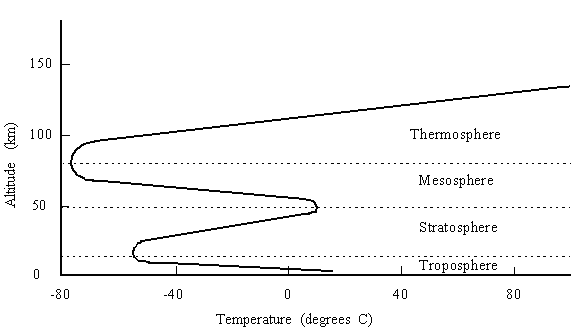# Atmospheric Pressure

The pressure on a scuba diver's body is the gravitational force per unit area due to the water and air above him. Because the density of water is nearly constant, varying only slightly with temperature, its contribution to the total pressure is easy to calculate: it is simply the product of the water density, the length of the water column above the diver, and the gravitational acceleration. The atmospheric contribution, on the other hand, is more difficult to determine because the density of the air varies significantly with altitude. When diving above sea level, the column of air above the diver is shorter, and its contribution to the total pressure felt by the diver is therefore smaller. Decompression tables and algorithms must take this into account.

`dP = - Dg dz                     Equation 1`

`PV = RT                          Equation 2`

`D = M/V                          Equation 3`

`dP = - (Mg/V)dz                  Equation 4`

`dP/P = -Mg/RT                    Equation 5`Accordingly, below the tropopause

`T = T0 - Bz                       Equation 6`

`dP/P = - (Mg/R)/(T0 -Bz)          Equation 7`

`P(z)/P0 = [1 - z(B/T0)]^(Mg/BR)   Equation 8`

`P(z)/P0 = (1 - 0.02255z)^5.256    Equation 9`

```           z(km)  P/P0
0	   1
0.5	  0.942
1.0	  0.887
1.5	  0.834
2.0	  0.785
2.5	  0.737
3.0	  0.692```
```
```
REFERENCES: